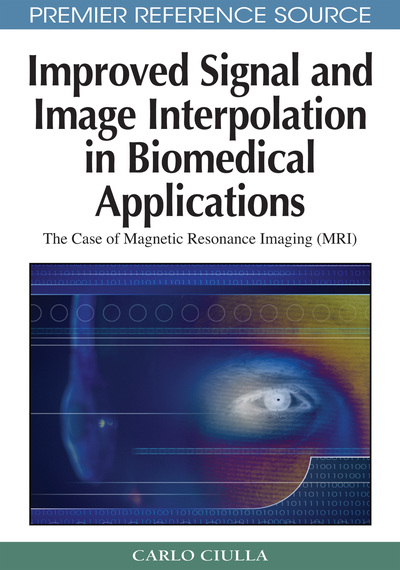# The Unifying Theory Embraces Lagrange and Sinc Interpolation Functions

Carlo Ciulla (Lane College, USA)
DOI: 10.4018/978-1-60566-202-2.ch018

## Abstract

This chapter is devoted to the mathematics of the Lagrange and Sinc SRE-based interpolation functions. The organization of the text of this chapter is consistent with that of chapters VII, X, and XIV. The basic aim of this chapter is to employ the methodology outlined in the book such to develop a mathematical formulation that allows interpolation error improvement also for Lagrange and Sinc interpolation functions. This is achieved through two instruments that bridge classic interpolation with the present innovative theory. The instruments are the Intensity-Curvature Functional (?E) and the Sub-pixel Efficacy Region (SRE). Math processes are thus presented that start from the calculation of the intensitycurvature terms and the corresponding Intensity-Curvature Functional, determine the SRE and employ the formula of the unifying theory (see equations  and  for Lagrange and Sinc respectively) to calculate the novel re-sampling locations for the two model interpolation functions. A section of this chapter is delegated to recall to the reader the characterization of upper and lower bounds of interpolation error improvement and interpolation error respectively. Details of this section are reported elsewhere (Ciulla & Deek, 2006). Finally the theoretical presentation of resilient interpolation is extended also to Lagrange and Sinc as it was already presented for the two linear functions and the two B-Splines that were object of treatise in Parts II, III and IV of this book. Although the logic behind the math of resilient interpolation is explained and characterized, resilient interpolation remains in this book a theoretical conceptualization which looks forward to empirical confirmation.
Chapter Preview
Top

## Calculation Of The Lagrange Intensity-Curvature Terms Before And After Interpolation

Let Eo and EIN be the intensity-curvature terms before and after interpolation. While the full math process employed for their calculation is reported in appendix VII, this paragraph illustrates the two resulting formulations:

## Complete Chapter List

Search this Book:
Reset